×

Search anything:

# Face Aging using Conditional GANs with Keras implementation

#### Machine Learning (ML) GANsFREE BOOK -> Problems for the day before your Coding Interview (on Amazon)

Reading time: 50 minutes | Coding time: 25 minutes

Face Aging, a.k.a. age synthesis and age progression can be defined as aesthetically rendering an image of a face with natural aging and rejuvenating effects on the individual face.

Traditional face aging approaches can be split into 2 types:

• prototyping
• modeling

### Prototyping approach

➤ Estimate average faces within predefined age groups

➤ The discrepancies between these faces constitute the aging patterns which are then used to transform an input face image into the target age group.

Pros - Are simple and fast

Cons - As they are based on general rules, they totally discard the personalized info which often results in unrealistic images.

### Modeling approach

➤ Employ parametric models to simulate the aging mechanisms of muscles, skin and skull of a particular individual

Both these approaches generally require face aging sequences of the same person with wide range of ages which are very costly to collect.

These approaches are helpful when we want to model the aging patterns sans natural human facial features (its personality traits, facial expression, possible facial accessories, etc.)

Anyway, in most of the real-life use cases, face aging must be combined with other alterations to the face, e.g. adding sunglasses or moustaches.

Such non-trivial modifications call for global generative models of human faces.

This is where the Age-cGAN model comes in.

You see, vanilla GANs are explicitly trained to generate the most realistic and credible images which should be hard to distinguish from real data.

Obviously, since their inception, GANs have been utilized to perform modifications on photos of human faces, viz. changing the hair colour, adding spectacles and even the cool application of binary aging (making the face look younger or older without using particular age categories to classify the face into).

But a familiar problem of these GAN-based methods for face modification is that, the original person's identity is often lost in the modified image.

The Age-cGAN model focuses on identity-preserving face aging.

The original paper made 2 new contributions to this field:

1. The Age-cGAN (Age Conditional GAN) was the first GAN to generate high quality artificial images within defined age categories.

2. The authors proposed a novel latent vector optimization approach which allows Age-cGAN to reconstruct an input face image, preserving the identity of the original person.

## Introducing Conditional GANs for Face Aging

Conditional GANs (cGANs) extend the idea of plain GANs, allowing us to control the output of the generator network.

We know that face aging involves changing the face of a person, as the person grows older or younger, making no changes to their identity.

In most other models (GANs included), the identity or appearance of a person is lost by 50% as facial expressions and superficial accessories, such as spectacles and facial hair, are not taken into consideration.

Age-cGANs consider all of these attributes, and that's why they are better than simple GANs in this aspect.

## Understanding cGANs

Read this to get an intuitive introduction of GANs.

GANs can be extended to a conditional both provided both the generator and discriminator networks are conditioned on some extra information, y.

y can be any kind of additional information, including class labels and integer data.

In practice, y can be any information related to the target face image - facial pose, level of illumination, etc.

The conditioning can be performed by feeding y into both the generator and discriminator as an additional input layer.

Some drawbacks of vanilla GANS:

1. Users have no control over the category of the images generated. When we add a condition y to the generator, we can only generate images of a specific category, using y.

2. Thus, they can learn only 1 category and it's highly tedious to construct GANs for multiple categories.

A cGAN overcomes these difficulties and can be used to generate multi-modal models with different conditions for various categories.

Below is the architecture of a cGAN: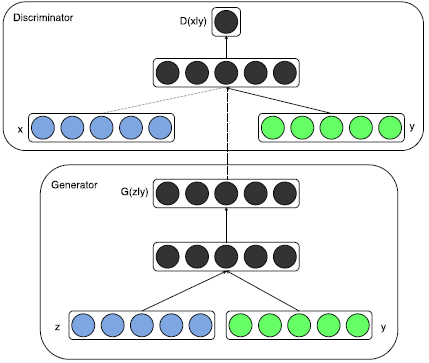Let us understand the working of a cGAN from this architecture -

In the generator, the prior input noise ${p}_{z}\left(z\right)$, and y are combined to give a joint hidden representation, the adversarial training framework making it easy to compose this hidden representation by allowing a lot of flexibility.

In the discriminator, x and y are presented as inputs and to a discriminative function (represented technically by a Multilayer Perceptron).

The objective function of a 2-player minimax game would be as

Here, G is the generator network and D is the discriminator network.

The loss for the discriminator is and the loss for the generator is .

$G\left(z|y\right)$ is modeling the distribution of our data given z and y.

z is a prior noise distribution of a dimension 100 drawn from a normal distribution.

## Architecture of the Age-cGAN

The Age-cGAN model proposed by the authors in the original paper used the same design for the Generator G and the Discriminator D as in Radford, et al. (2016), "Unsupervised representation learning with deep convolutional generative adversarial networks", the paper which introduced DCGANs.

In adherence to Perarnau, et al. (2016), "Invertible conditional GANs for image editing", the authors add the conditional information at the input of G and at the first convolutional layer of D.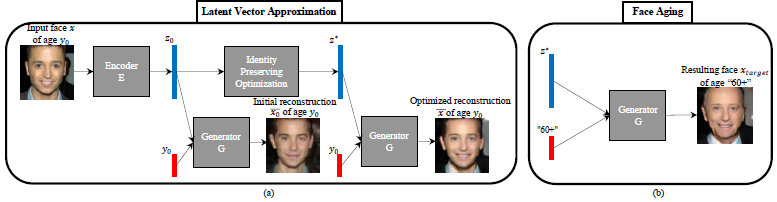The picture shows the face aging method used in Age-cGANs.

a) depicts an approximation of the latent vector to reconstruct the input image

b) illustrates switching the age condition at the input of the generator G to perform face aging.

Diving deeper into more technical details, now.

The Age-cGAN consists of 4 networks - an encoder, the FaceNet, a generator network and a discriminator network.

The functions of each of the 4 networks -

a) Encoder - Helps us learn the inverse mapping of input face images and the age condition with the latent vector ${z}_{0}$.

b) FaceNet - It is a face recognition network which learns the difference between an input image x and a reconstructed image $\stackrel{~}{x}$.

c) Generator network - Takes a hidden (latent) representation consisting of a face image and a condition vector, and generates an image.

d) Discriminator network - Discriminates between the real and fake images.

cGANs have a little drawback - they can't learn the task of inverse mapping an input image x with attributes y to a latent vector z, which is necessary for the image reconstruction: .

To solve this problem, we use an encoder network, which we can train to approximate the inverse mapping of input images x.

## A) The encoder network

1. Its main purpose is to generate a latent vector of the provided images, that we can use to generate face images at a target age.
2. Basically, it takes an image of a dimension of (64, 64, 3) and converts it into a 100-dimensional vector.
3. The architecture is a deep convolutional neural network (CNN) - containing 4 convolutional blocks and 2 fully connected (dense) layers.
4. Each convolutional block, a.k.a. Conv block contains a convolutional layer, a batch normalization layer, and an activation function.
5. In each Conv block, each Conv layer is followed by a batch normalization layer, except the first Conv layer.

## B) The generator network

1. The primary objective is to generate an image having the dimension (64, 64, 3).
2. It takes a 100-dimensional latent vector (from the encoder) and some extra information y, and tries to generate realistic images.
3. The architecture here again, is a deep CNN made up of dense, upsampling, and Conv layers.
4. It takes 2 input values - a noise vector and a conditioning value.
5. The conditioning value is the additional information we'll provide to the network, for the Age-cGAN, this will be the age.

## C) The discriminator network

1. What the discriminator network does is identify whether the provided image is fake or real. Simple.
2. It does this by passing the image through a series of downsampling layers and some classification layers, i.e. it predicts whether the image is fake or real.
3. Like the 2 networks before, this network is another deep CNN.
4. It comprises several Conv blocks - each containing a Conv layer, a batch normalization layer, and an activation function, except the first Conv block, which lacks the batch normalization layer.

## D) Face recognition network

1. The FaceNet has the main task of recognizing a person's identity in a given image.
2. To build the model, we will be using the pre-trained Inception-ResNet-v2 model without the fully connected layers.

To learn more about the Inception-ResNet-v2 model, you could also read the original paper by Szegedy, et al. (2016), "Inception-v4, Inception-ResNet and the Impact of Residual Connections on Learning".

1. The pretrained Inception-ResNet-v2 network, once provided with an image, returns the corresponding embedding.
2. The extracted embeddings for the real image and the reconstructed image can be calculated by calculating the Euclidean distance of the embeddings.
3. The Face Recognition (FR) network generates a 128-dimensional embedding of a particular input fed to it, to improve the genrator and the encoder networks.

## Stages of the Age-cGAN

The Age-cGAN model has multiple stages of training. The Age-cGAN has 4 networks, which get trained as 3 stages which are as follows:

1) Conditional GAN training
2) Initial latent vector optimization
3) Latent vector optimization

## A) Conditional GAN Training

➤ This is the first stage in the training of a conditional GAN.
➤ In this stage, we train both the generator and the discriminator networks.
➤ Once the generator network is trained, it can generate blurred images of a face.
➤ This stage is comparable to training a vanilla GAN, where we train both the networks simultaneously.

### ➔ The training objective function

The training objective function for the training of cGANs can be represented as follows:

Does the above equation seem familiar to you? You're right!
This equation is identical to the equation of the objective function of a 2-player minimax game we have seen before.

Training a cGAN network entails optimizing the function .

Training a cGAN can ideally be thought of as a minimax game, in which both the generator and the discriminator networks are trained simultaneously.

In the preceding equation,

1. ${\theta }_{G}$ represents the parameters of the generator network.
2. ${\theta }_{D}$ represents the parameter of the discriminator network.
3. is the loss for the discriminator model.
4. is the loss for the generator model, and
5. ${p}_{data}$ is the distribution of all possible images.

## B) Initial latent vector approximation

➤ Initial latent vector approximation is a method to estimate a latent vector to optimize the reconstruction of face images.
➤ To approximate a latent vector, we have an encoder network.
➤ We train the encoder network on the generated images and real images.
➤ Once trained, it will start generating latent vectors from the learned distribution.
➤The training objective function for training the encoder network is the Euclidean distance loss.

Although GANs are no doubt one of the most powerful generative models at present, they cannot exactly reproduce the details of all real-life face images with their infinite possibilities of minor facial details, superficial accessories, backgrounds, etc.

It is common fact that, a natural input face image can be rather approximated than exactly reconstructed with Age-cGAN.

## C) Latent vector optimization

➤ During latent vector optimization, we optimize the encoder network and the generator network simultaneously.
➤ The equation used for this purpose is as follows:

This equation follows the novel "Identity Preserving" latent vector optimization approach followed by the authors in their paper.

The key idea is simple - given a face recognition neural network FR which is able to recognize a person's identity in an input face image x, the difference between the identities in the original and reconstructed images x and $\stackrel{~}{x}$ can be expressed as the Euclidean distance between the corresponding embeddings FR(x) and FR($\stackrel{~}{x}$).

Hence, minimizing this distance should improve the identity preservation in the reconstructed image $\stackrel{~}{x}$.

Here, ${z}_{IP}^{*}$ denotes the identity-preserving latent vector optimization.

FR stands for Face Recognition network, which is an internal implementation of the FaceNet CNN.

The equation above indicates that the Euclidean distance between the real image and the reconstructed images should be minimal.

To sum it up, in this stage, we try to minimize the distance in order to maximize identity preservation.

✪ The first step of the face aging method presented in the paper (i.e. input-face reconstruction) is the key one.

✪ Details of Age-cGAN training from the original paper -
➢ The model was optimized using the Adam algorithm, and was trained for 100 epochs.
➢ In order to encode the person's age, the authors had defined 6 age categories: 0-18, 19-29, 30-39, 40-49, 50-59 and 60+ years old.
➢ The age categories were selected so that the training dataset contained at least 5,000 examples in each age category.

# Experiments on the Age-cGAN model

The authors trained the Age-cGAN on the IMDB-Wiki_cleaned  dataset containing around 120,000 images, which is a subset of the public IMDB-Wiki dataset .

110,000 images were used for training of the Age-cGAN model and the remaining 10,000 were used for the evaluation of identity-preserving face reconstruction.

## A) Age-Conditioned Face Generation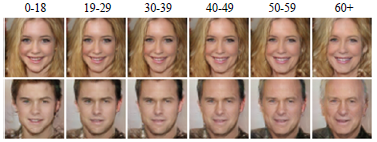The above image illustrates examples of synthetic images generated by the Age-cGAN model using 2 random latent vectors z (rows), conditioned on the respective age categories y (columns).

It is observed that the Age-cGAN model perfectly disentangles image information encoded by latent vectors z and by conditions y, making them independent.

Additionally, the latent vectors z encode the person's identity - hair style, facial attributes, etc., while y uniquely encodes the age.

### ➔ Steps taken to measure performance of the model

I To measure how well the Age-cGAN model manages to generate faces belonging to precise age-categories, a state-of-the-art age estimation CNN model  was employed.

II The performances of the age estimation CNN (on real images from the test part of the IMDB-Wiki_cleaned dataset) and Age-cGAN (10,000 synthetic images generated) was compared.

### ➔ Observations & Results

➢ Despite the age estimation CNN had never seen synthetic images during the training, the resulting mean age estimation accuracy on synthetic images is just 17% lower than on natural ones.

➢ This shows that the Age-cGAN model can be used for the production of realistic face images with the required age.

## B) Identity-Preserving Face Reconstruction and Aging

The authors performed face reconstruction in 2 iterations:

I. Using initial latent approximations obtained from the encoder E and then
II. Using optimized latent approximations obtained by either "Pixelwise" (${z}_{pixel}^{✱}$) or "Identity-Preserving" (${z}_{IP}^{*}$) optimization approaches.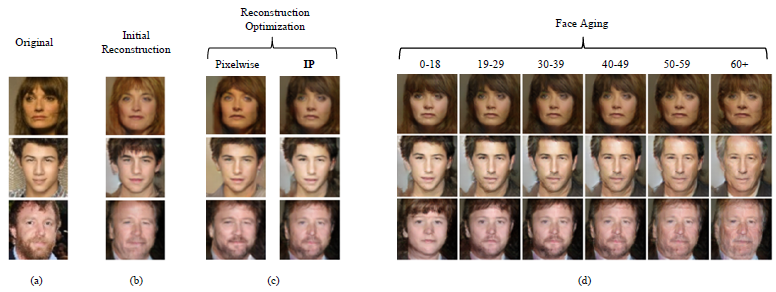The image above shows some examples of face reconstruction and aging.

a) depicts the original test images.

b) illustrates the reconstructed images generated using the initial latent approximations: ${z}_{0}$

c) demonstrates the reconstructed images generated using the "Pixelwise" and "Identity-Preserving" optimized latent approximations: ${z}_{pixel}^{✱}$ and ${z}_{IP}^{✱}$, and

d) shows the aging of the reconstructed images generated using the identity-preserving ${z}_{IP}^{✱}$ latent approximation and conditioned on the respective age categories y (one per column)

It can be clearly noted from the above picture that the optimized reconstructions are closer to the original images than the initial ones.

But things get more complicated when it comes to the comparison of the 2 latent vector optimization approaches.

● The "Pixelwise" optimization shows the superficial face details (hair color, beard line, etc.) better
● The "Identity-Preserving" optimization represents the identity traits (like the form of the head or the form of the eyes) better.

### ➔ Steps taken to measure performance of the model

I) For the objective comparison of the 2 approaches for the identity-preserving face reconstruction, the authors used the "OpenFace"  software, which is one of the renowned open-source face recognition algorithms.

II. Given 2 face images, "OpenFace" decides whether they belong to the same person or not.

III. Using both the approaches, 10,000 test images of the IMDB-Wiki_cleaned dataset were reconstructed and the resulting images alongside the corresponding original ones were then fed to "OpenFace".

### ➔ Observations & Results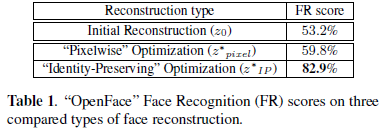➢ Table 1 presents the percentages of "OpenFace" positive outputs (i.e. when the software believed that a face image and its reconstruction belonged to the same person).

The results confirm the visual observations presented above.

➢ Initial reconstructions allow "OpenFace" to recognize the original person in only half of the test examples.

➢ This number is slightly increased by "Pixelwise" optimization but the improvement is very slight.

➢ Conversely, "Identity-Preserving" optimization approach preserves the individual's identities far better, giving the best face recognition performance of 82.9%.

Now we shall cover the basic implementation of all the 4 networks - encoder, generator, discriminator and face recognition - using the Keras library.

Excited? I am!

## Implementation of the networks in Keras

The complete implementation of the Age-cGAN model is too huge (~600 lines of code) to be demonstrated in one post, so I decided to show you how to build the networks, the crucial components of the model, in Keras.

Let's import all the required libraries first:

``````import os
import time
import matplotlib.pyplot as plt
import numpy as np
import tensorflow as tf

from datetime import datetime
from keras import Input, Model
from keras.applications import InceptionResNetV2
from keras.callbacks import TensorBoard
from keras.layers import Conv2D, Flatten, Dense, BatchNormalization
from keras.layers import Reshape, concatenate, LeakyReLU, Lambda
from keras.layers import K, Activation, UpSampling2D, Dropout
from keras.utils import to_categorical
from keras_preprocessing import image
``````

## A) Encoder network

``````def build_encoder():

"""
Encoder Network
"""

input_layer = Input(shape = (64, 64, 3))

## 1st Convolutional Block
enc = Conv2D(filters = 32, kernel_size = 5, strides = 2, padding = 'same')(input_layer)
enc = LeakyReLU(alpha = 0.2)(enc)

## 2nd Convolutional Block
enc = Conv2D(filters = 64, kernel_size = 5, strides = 2, padding = 'same')(enc)
enc = BatchNormalization()(enc)
enc = LeakyReLU(alpha = 0.2)(enc)

## 3rd Convolutional Block
enc = Conv2D(filters = 128, kernel_size = 5, strides = 2, padding = 'same')(enc)
enc = BatchNormalization()(enc)
enc = LeakyReLU(alpha = 0.2)(enc)

## 4th Convolutional Block
enc = Conv2D(filters = 256, kernel_size = 5, strides = 2, padding = 'same')(enc)
enc = BatchNormalization()(enc)
enc = LeakyReLU(alpha = 0.2)(enc)

## Flatten layer
enc = Flatten()(enc)

## 1st Fully Connected Layer
enc = Dense(4096)(enc)
enc = BatchNormalization()(enc)
enc = LeakyReLU(alpha = 0.2)(enc)

## 2nd Fully Connected Layer
enc = Dense(100)(enc)

## Create a model
model = Model(inputs = [input_layer], outputs = [enc])
return model
``````

➤ The Flatten() layer converts an n-dimensional tensor to a one-dimensional tensor (array). The process is known as flattening.

## B) Generator network

``````def build_generator():

"""
Generator Network
"""

latent_dims = 100
num_classes = 6

input_z_noise = Input(shape = (latent_dims, ))
input_label = Input(shape = (num_classes, ))

x = concatenate([input_z_noise, input_label])

x = Dense(2048, input_dim = latent_dims + num_classes)(x)
x = LeakyReLU(alpha = 0.2)(x)
x = Dropout(0.2)(x)

x = Dense(256 * 8 * 8)(x)
x = BatchNormalization()(x)
x = LeakyReLU(alpha = 0.2)(x)
x = Dropout(0.2)(x)

x = Reshape((8, 8, 256))(x)

x = UpSampling2D(size = (2, 2))(x)
x = Conv2D(filters = 128, kernel_size = 5, padding = 'same')(x)
x = BatchNormalization(momentum = 0.8)(x)
x = LeakyReLU(alpha = 0.2)(x)

x = UpSampling2D(size = (2, 2))(x)
x = Conv2D(filters = 64, kernel_size = 5, padding = 'same')(x)
x = BatchNormalization(momentum = 0.8)(x)
x = LeakyReLU(alpha = 0.2)(x)

x = UpSampling2D(size = (2, 2))(x)
x = Conv2D(filters = 3, kernel_size = 5, padding = 'same')(x)
x = Activation('tanh')(x)

model = Model(inputs = [input_z_noise, input_label], outputs = [x])
return model
``````

➤ The UpSampling2D() function is for the process of repeating the rows a specified number of times x and repeating the columns a specified number of times y, respectively.

Upsampling is a technique to keep the dimension of the output the same as before, while reducing the computation per layer simultaneously.

## C) Discriminator network

``````def expand_label_input(x):
x = K.expand_dims(x, axis = 1)
x = K.expand_dims(x, axis = 1)
x = K.tile(x, [1, 32, 32, 1])
return x
``````
``````def build_discriminator():

"""
Discriminator Network
"""

input_shape = (64, 64, 3)
label_shape = (6, )
image_input = Input(shape = input_shape)
label_input = Input(shape = label_shape)

x = Conv2D(64, kernel_size = 3, strides = 2, padding = 'same')(image_input)
x = LeakyReLU(alpha = 0.2)(x)

label_input1 = Lambda(expand_label_input)(label_input)
x = concatenate([x, label_input1], axis = 3)

x = Conv2D(128, kernel_size = 3, strides = 2, padding = 'same')(x)
x = BatchNormalization()(x)
x = LeakyReLU(alpha = 0.2)(x)

x = Conv2D(256, kernel_size = 3, strides = 2, padding = 'same')(x)
x = BatchNormalization()(x)
x = LeakyReLU(alpha = 0.2)(x)

x = Conv2D(512, kernel_size = 3, strides = 2, padding = 'same')(x)
x = BatchNormalization()(x)
x = LeakyReLU(alpha = 0.2)(x)

x = Flatten()(x)
x = Dense(1, activation = 'sigmoid')(x)

model = Model(inputs = [image_input, label_input], outputs = [x])
return model
``````

➤ The expand_label_input() function helps convert the shape of the label input from (6, ) to (32, 32, 6).

## D) Face Recognition Network

``````def build_fr_model(input_shape):

resnet_model = InceptionResNetV2(include_top = False, weights = 'imagenet',
input_shape = input_shape, pooling = 'avg')
image_input = resnet_model.input
x = resnet_model.layers[-1].output
out = Dense(128)(x)
embedder_model = Model(inputs = [image_input], outputs = [out])

input_layer = Input(shape = input_shape)

x = embedder_model(input_layer)
output = Lambda(lambda x: K.l2_normalize(x, axis = -1))(x)

model = Model(inputs = [input_layer], outputs = [output])
return model
``````

### Bonus: The entire code can be found at this Google Colab link and this repository

Finally, let's have a look at some cool applications of the Age-cGAN model.

## I. Cross-age face recognition

➤ This can be incorporated into security applications as means of authorization e.g. smartphone unlocking or desktop unlocking.

➤ Current face recongnition system suffer from the problem of necessary updation with passage of time.

➤ With Age-cGAN models, the lifespan of cross-age face recognition systems will be much longer.

## II. Finding lost children

➤ As the age of a child increases, their facial features change, and it becomes much harder to identify them.

➤ An Age-cGAN model can simulate a person's face at a specified age easily.

## III. Entertainment

➤ For e.g. in mobile applications, to show and share a person's pictures at a specified age.

The famous phone application FaceApp is the best example for this use case.

## IV. Visual effects in movies

➤ It requires lots of visual effects and to manually simulate a person's face when they are older. This is a very tedious and lengthy process too.

➤ Age-cGAN models can speed up this process and reduce the costs of creating and simulating faces significantly.

 Radford, et al. (2016), "Unsupervised representation learning with deep convolutional generative adversarial networks"
 Perarnau, et al. (2016), "Invertible conditional GANs for image editing"
 Antipov, et al. (2016), "Apparent age estimation from face images combining general and children-specialized deep learning models"
 Rothe, et al. (2015), "DEX: Deep EXpectation of apparent age from a single image"
 Ludwiczuk, et al. (2016), "Openface: A general-purpose face recognition library with mobile applications"
 Szegedy, et al. (2016), "Inception-v4, Inception-ResNet and the Impact of Residual Connections on Learning"
 Antipov, et al. (2017), "Face Aging with Conditional Generative Adversarial Networks"
 Mirza, et al. (2014), "Conditional Generative Adversarial Nets"